Tamilnadu State Board New Syllabus Samacheer Kalvi 12th Maths Guide Pdf Chapter 3 Theory of Equations Ex 3.5 Textbook Questions and Answers, Notes.

## Tamilnadu Samacheer Kalvi 12th Maths Solutions Chapter 3 Theory of Equations Ex 3.5

Question 1.
Solve the following equations
(i) sin² x – 5 sin x + 4 = 0
Solution:
sin2x – 5sinx + 4 = 0
Let y = sin x
(y2 – 5y + 4 = 0
(y – 1) (y – 4) = 0
(y – 1) = o or (y – 4) = 0
y = 1 or y = 4
sin x = 1 or sin x = 4 [not possible since sin x ≤ 1]
sin x = sin $$\frac{\pi}{2}$$
x = nπ + (-1)n α, n ∈ z.
x = nπ + (-1)n $$\frac{\pi}{2}$$

(ii) 12x³ + 8x = 29x² – 4 = 0
Solution:
12x³ – 29x² + 8x + 4 = 0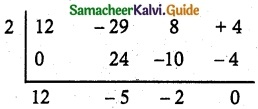12x² – 5x – 2 = 0
12x² – 8x + 3x – 2 = 0
4x(3x – 2) + 1(3x – 2) = 0
(3x – 2)(4x + 1) = 0
3x = 2, 4x = -1
x = $$\frac{2}{3}$$ or x = –$$\frac{1}{4}$$
The roots are 2, $$\frac{2}{3}$$, –$$\frac{1}{4}$$.Question 2.
Examine for the rational roots of
(i) 2x³ – x² – 1 = 0
Solution:
Sum of co-efficients = 2 – 1 – 1 = 0
⇒ x = 1 is a factor.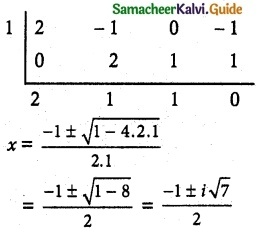Which is imaginary root
∴ x = 1 is rational root.

(ii) x8 – 3x + 1 = 0
Solution:
an = 1; a0 = 1
If $$\frac{p}{q}$$ is a root of the polynomial. (p, q) = 1
By rational root theorem, it has no rational roots.Question 3.
Solve: 8x$$\frac{3}{2n}$$ – 8x$$\frac{-3}{2n}$$ = 63.
Solution:
Put k = $$\frac{3}{2n}$$ ∴ 8xk – 8x-k = 63
8xk – $$\frac{8}{x^k}$$ = 63
8x2k – 8 = 63xk
8x2k – 63xk – 8 = 0
(xk – 8) (8xk + 1) = 0
xk – 8 = 0
xk = 8
x$$\frac{3}{2n}$$ = 8
x = 8$$\frac{3}{2n}$$ (2³)$$\frac{3}{2n}$$ = (2²)n = 4n
∴ x = 4n is a root of the equation.

Question 4.
Solve: 2$$\sqrt{\frac{x}{a}}$$ + 3$$\sqrt{\frac{a}{x}}$$ = $$\frac{b}{a}$$ = $$\frac{6a}{b}$$
Solution:
put $$\sqrt{\frac{x}{a}}$$ = y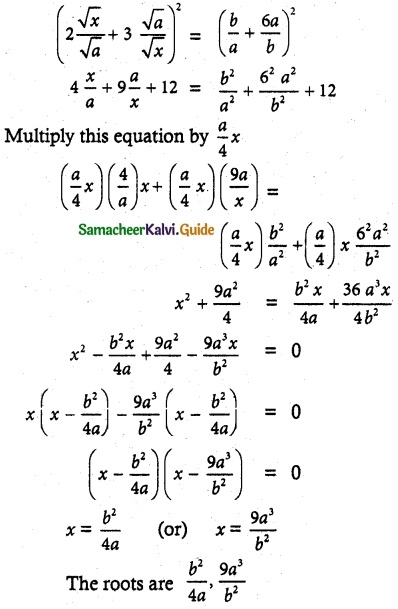Question 5.
Solve the equations
(i) 6x4 – 35x³ + 62x² – 35x + 6 = 0
Solution:
This is Type I even degree reciprocal equation. Hence it can be rewritten as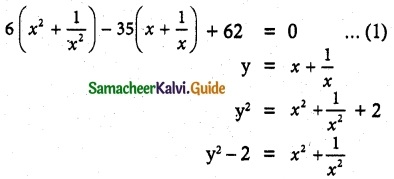(1) ⇒ 6(y² – 2) – 35y + 62 = 0
6y² – 12 – 35y + 62 = 0
6y² – 35y + 50 = 0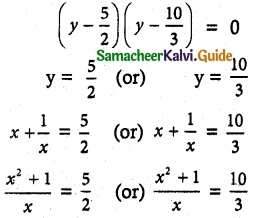2x² + 2 – 5x = 0 (or) 3x² + 3 = 10x
2x² – 5x + 2 = 0 (or) 3x² – 10x + 3 = 0
x = 2, $$\frac{1}{2}$$ (or) x = 3, $$\frac{1}{3}$$
Roots are 2, $$\frac{1}{2}$$, 3 and $$\frac{1}{3}$$(ii) x4 + 3x³ – 3x – 1 = 0
Solution:
(x – 1) and (x + 1) is a factor.(x – 1)(x + 1)(x² + 3x + 1) = 0
x – 1 = 0 (or) x + 1 = 0 (or) x² + 3x + 1 = 0
x = 1 (or) x = -1 (or) x² + 3x = -1Question 6.
Find all real numbers satisfying
Solution:
4x – 3 (2x+2) + 25 = 0
⇒ (22)x – 3(2x . 22) + 25 = 0
(22)x – 12 . 2x+ 32 = 0
Let y = 2x
y2 – 12y + 32 = 0
⇒ (y – 4) (y – 8) = 0
y – 4 = 0 or y – 8 = 0
Case (i): 2x = 4
⇒ 2x = (2)2
⇒ x = 2
Case (ii): 2x = 8
⇒ 2x = (2)3
⇒ x = 3
∴ The roots are 2, 3Question 7.
Solve the equation 6x4 – 5x³ – 38x² – 5x + 6 = 0 if it is known that $$\frac{1}{3}$$ is a solution.
Solution:
6x4 – 5x³ – 38x² – 5x + 6 = 06(y² – 2) – 5y – 38 = 0
6y² – 12 – 5y – 38 = 0
6y² – 5y – 50 = 0
6y² – 20y + 15y  – 50 = 0
2y(3y – 10) + 5(3y – 10) = 0
(3y – 10)(2y + 5) = 0
3y = 10, 2y = -5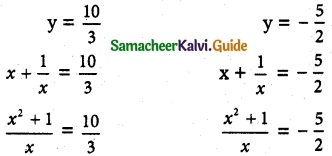3x² + 3 = 10x, 2x² + 2 = -5x
3x² – 10x + 3 = 0, 2x² + 5x + 2 = 0
(x – 3)(3x – 1) = 0, (2x + 1)(x + 1) = 0
x = 3, x = $$\frac{1}{3}$$ or x = $$\frac{-1}{2}$$, x= -2
The roots are 3, $$\frac{1}{3}$$, -2, $$\frac{-1}{2}$$.